# Python tkinter place ( layout )

Tkinter Grid Layout Pack

Positioning of widgets based on absolute and relative coordinates with respect to parent window.
Tkinter layout management using place for absolute & relative height width and positioning of widget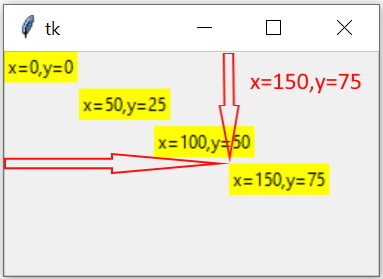Here is an example of layout of the page to place the components using x , y coordinates.

We will keep Labels showing x ( horizontal ) and y ( vertical ) positions

Here `x=0` is left edge and `y=0` is top edge of the window.
``````import tkinter as tk
my_w = tk.Tk()
my_w.geometry("250x150")  # Size of the window

l1=tk.Label(my_w,text='x=0,y=0',bg='yellow')
l1.place(x=0,y=0)

l2=tk.Label(my_w,text='x=50,y=25',bg='yellow')
l2.place(x=50,y=25)

l3=tk.Label(my_w,text='x=100,y=50',bg='yellow')
l3.place(x=100,y=50)

l4=tk.Label(my_w,text='x=150,y=75',bg='yellow')
l4.place(x=150,y=75)

my_w.mainloop()  # Keep the window open``````

## relx & rely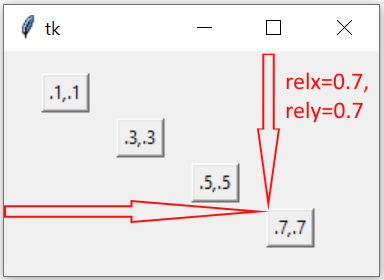We can use relative x ( horizontal ) and y ( vertical ) position with respect to width and height of the window by using relx and rely options.
relx and rely can take values from 0 to 1
`relx=0` is left edge of the window `relx=1` is right edge of the window.

`rely=0` is top of the window and `rely=1` is the bottom edge of the window.
Here default value for `anchor='nw'` is used.
``````l1=tk.Label(my_w,text='relx=.1,rely=.1 ',bg='yellow')
l1.place(relx=.1,rely=.1)

l2=tk.Label(my_w,text='x=.3,y=.3',bg='yellow')
l2.place(relx=.3,rely=.3)

l3=tk.Label(my_w,text='x=.5,y=.5',bg='yellow')
l3.place(relx=.5,rely=.5)

l4=tk.Label(my_w,text='x=.7,y=.7',bg='yellow')
l4.place(relx=.7,rely=.7)``````

## Understanding anchor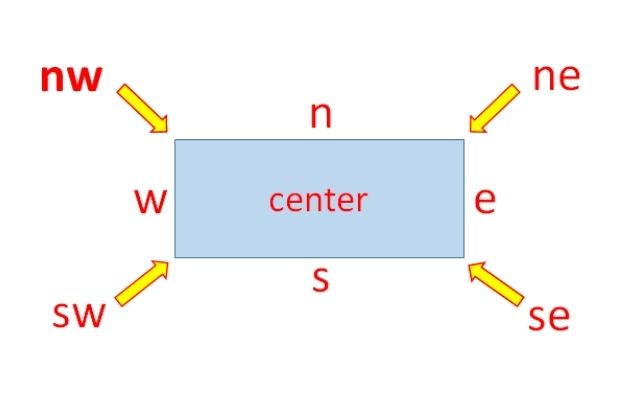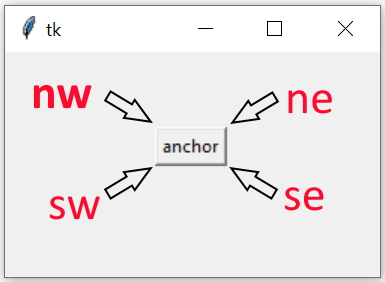The option anchor says which side or the corner of the widget to be considered for positing the widget in layout.
Values of anchor option is here

`anchor=nw(default),ne,se,sw,n,s,e,w,center`

## Using relx & rely with anchor at four corners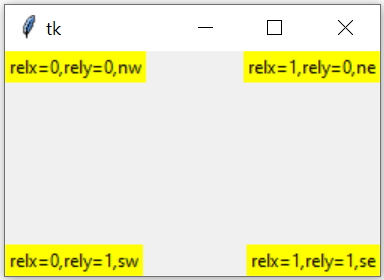We will place four Labels at four corners of a window. We will use relx and rely with values either 0 or 1 to assign relative position to widgets.

We have to change the anchor values to position the widget at corners. Note that we have not used any fraction values for relx or rely.
``````l1=tk.Label(my_w,text='x=0, y=0, nw',bg='yellow')
l1.place(relx=0,rely=0,anchor='nw') # top left

l2=tk.Label(my_w,text='x=1, y=0, ne',bg='yellow')
l2.place(relx=1,rely=0,anchor='ne') # top right

l3=tk.Label(my_w,text='x=1, y=1, se',bg='yellow')
l3.place(relx=1,rely=1,anchor='se') # bottom right

l4=tk.Label(my_w,text='x=0, y=1, sw',bg='yellow')
l4.place(relx=0,rely=1,anchor='sw') # bottom left ``````

## anchor and x,y coordinates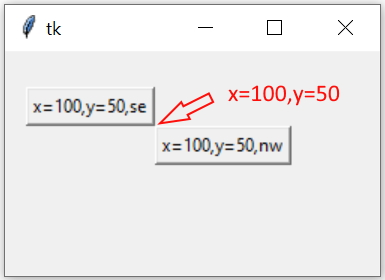Here both the buttons has same x and y coordinate value but are placed at different positions due to their respective anchor values. Note that the bottom right ( `anchor='se'` ) corner of first widget is touching the top left ( `anchor='nw'` ) of second widget.
``````b1=tk.Button(my_w,text='x=100,y=50,se')
b1.place(x=100,y=50,anchor='se') # top left

b2=tk.Button(my_w,text='x=100,y=50,nw')
b2.place(x=100,y=50,anchor='nw')``````

## relwidth & relheight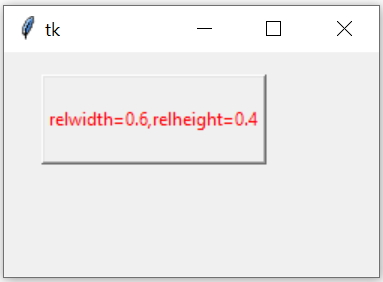The dimension of the widget can be fixed based on the size of the parent window by using relwidth and relheight options.

Here `relwidth=1` will occupy the full width of the parent window, similarly `relheight=1` will occupy the full height of the window.
``````import tkinter as tk
my_w = tk.Tk()
my_w.geometry("250x150")  # Size of the window

b1=tk.Button(my_w,text='relwidth=0.6,relheight=0.4',fg='red')
b1.place(relx=0.1,rely=0.1,relwidth=0.6,relheight=0.4)

my_w.mainloop()  # Keep the window open``````

Subscribe to our YouTube Channel here

## Subscribe

* indicates required
Subscribe to plus2netplus2net.com